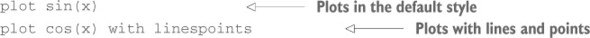# Chapter 3. The heart of the matter: the plot command

### This chapter covers

• Plotting functions and data
• Math with gnuplot
• Inline transformations
• Logarithmic plots
• Smoothing data

In gnuplot, you use the plot command to create plots of functions and data. It is, hands down, the most frequently used and most important of all gnuplot commands. In this chapter, we’ll take a systematic look at the plot command and the ways it can be used.

You’ll begin by making simple plots of functions and data. Then I’ll introduce inline transformations: gnuplot can apply arbitrary transformations to the data as it’s being plotted. You’ll learn how to use this useful feature after we take a quick detour to describe how to do math with gnuplot. Logarithmic plots are another indispensable technique in graphical analysis, and I’ll show you how and when to use them. (If you’ve never seen them before, you’ll find a sidebar giving you more background on them.) Finally, we’ll be ready to discuss gnuplot’s built-in features to simplify, smooth, and summarize data.

### 3.1. Plotting functions and data

You use the plot command to plot both functions and data. Let’s look at plotting functions first and then move on to plotting data.

#### 3.1.1. Plotting functions

To plot a function, you use the plot command followed by the function to plot and (optionally) any style directives you wish to use (see chapters 6 and 9 to learn about available styles and how to specify them):